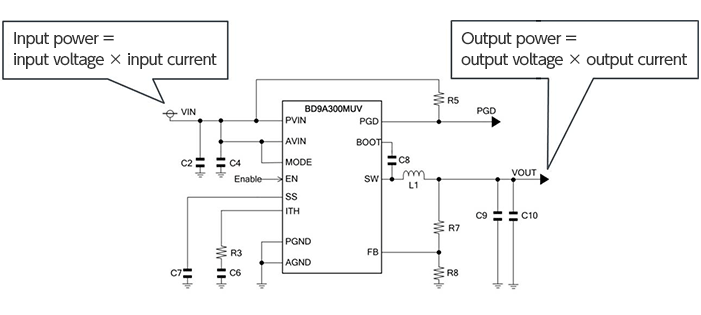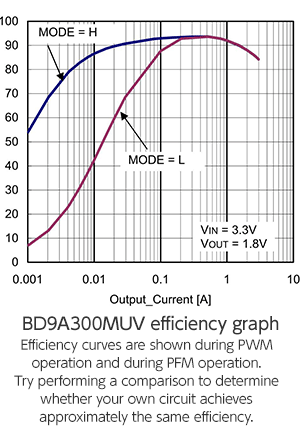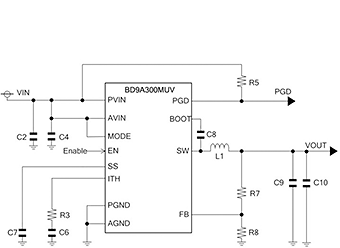Technical Information Site of Power Supply Design

2016.11.11 DC/DC

# Evaluating a Switching Regulator: Measurement of Efficiency

Characteristics and Evaluation Method of Switching Regulators

In the previous sections titled on [Evaluating a Switching Regulator], we discussed four topics [Output Voltage], [Load Regulation], [Load Response: Consideration and Measurement Method, and [Inductor Current Measurement]. Here we explain the fifth topic [Measurement of Efficiency].

Efficiency of a Switching Regulator

Before considering measurement of efficiency, we review the definition and meaning of the efficiency of a switching regulator.

The efficiency of a switching regulator is generally expressed as a percentage. There is no problem with using a decimal representation instead, but here we will use percentages. The efficiency is based on the following formula.As can be seen, there is nothing particularly remarkable here; the efficiency is simply the ratio of "the power that can be taken out" to "the power that is put in". For example, "90% efficiency" means that 90% of the input power can be used as output, with losses of 10%, primarily lost as heat. Switching regulators, that is, DC/DC converters and AC/DC converters, perform conversion of electric power, and so this should be easy to understand. Of course the efficiency of linear regulators such as LDO regulators, which perform voltage conversion, is also calculated in terms of power using the same formula.

Repeating everything again is probably not necessary, but as a reminder, the input power is the input voltage times the input current, and the output power is the output voltage times the output current. Hence it is obvious what needs to be measured in order to determine the efficiency.Measurements and Examination

Measurements are themselves comparatively simple. The average power values are measured, and calculations performed using the average power values. Ripples are inherent in the output of a switching regulator, and the output voltage and output current fluctuate due to ripples. There are cases in which ripples are also present in the input, but in any event, what is measured are the average values and not the peak values, which are affected by ripples. Measuring average power is not particularly difficult. If a voltmeter and an ammeter are used rather than an oscilloscope, then the measured values are themselves average values.

In addition, an output load is necessary. The steady-state average current is measured, and so an electronic load and resistance can be used.

Measurements are made using only a voltmeter and ammeter, but several measurements or more of the output load are made, including the minimum value, standard value, and maximum value of the feed circuit, and the like. In some cases, it may be necessary to plot the results on a graph. The input voltage is also varied over the anticipated range. Measurements are also made with the ambient temperature varied.

If an oscilloscope is used to monitor the input and output while performing measurements, the occurrence of any abnormal behavior caused by the load or the connected instruments can be determined. If oscillation or other phenomena are occurring, the average values measured by the voltmeter or ammeter cannot be regarded as data that can be used in evaluations. Moreover, when using an especially large load, care must be taken to ensure that there is no abnormal heat generation in ICs or other components. After confirming that sufficiently high efficiency is obtained at normal temperatures, measurements are performed at elevated temperatures, while controlling the temperature with some precision. If the Tj maximum rating is exceeded, abnormal behavior could occur even after problems with the circuit and operation have been eliminated, and in a worst-case scenario, failure could occur, so sufficient caution must be exercised.

When examining the measured efficiency, it is a good idea to first study a graph of the efficiency included on the data sheet. Often the circuit and components are based on a standard example of the data sheet, and so the efficiency curve will be fairly close to this curve. One method is to perform measurements under the same conditions as those used for the graph on the data sheet, to facilitate comparison. Comparison with an evaluation board supplied by the manufacturer is another worthwhile method. In this case, if external components and the like are different, it will be necessary to change the external components before measurements.

Important points are summarized below.• Efficiency is calculated using average power values (peak values, affected by ripples, are not used).
• Average values are obtained by using voltmeters and ammeters.
• Measurements are performed incorporating such variable factors as the anticipated input range, load (output) current, and ambient temperature.
• Performing measurements while using an oscilloscope for monitoring can be useful.
• During measurements with large loads, pay heed to abnormal behavior and heat generation.
• In evaluations, refer to data sheet efficiency graphs.

When Efficiency is Lower than Anticipated

When, as a result of examination, the conclusion that "there is no abnormal operation, but the efficiency is lower than expected" is reached, the reason for the lowered efficiency must be discovered, and adjustments made. To do this, if the location at which the loss is occurring and empirical rules for the components are also known in advance, measures can be taken in an effective and timely manner.

Losses occur in all parts where power is consumed within a circuit, but the major causes of losses are I2R losses, switching losses and self-consumption current losses, transition losses, and other losses.

I2R losses occur depending on the on resistance of internal power transistors and the series resistance value of external inductors. Hence checks are performed to ensure that the on resistance of power transistors and the series resistance of inductors are sufficiently low.

Switching losses and self-consumption current losses affect the current required for the gate driving current of the power transistors within the IC and the control circuit. For the IC with built-in power transistors, it is difficult to select the power MOSFET based on the gate charge. Accordingly, the ICs with small current consumption or component values that do not increase current consumption should be selected.

Transition losses occur because a high-side power MOSFET saturates in a short time during transitions. Dead time conduction losses are another known type of loss. These are fixed losses occurring within ICs, and in nearly all cases adjustment is not possible. Other losses amenable to adjustment include inductor core losses and board wiring resistances, but they represent only a very small fraction of all losses.

Thus there are a number of general points that can be made, but a large fraction of issues depend on the power supply IC used. Particularly for the IC with built-in power transistors, almost nothing can be done apart from confirming that external component values are appropriate. Of course power supply ICs are optimized and execute sophisticated control, and in effect ensure that the best possible characteristics can be obtained.

Conversely, we can conclude that it is extremely important to select an IC capable of delivering the target efficiency.

That said, it is not the case that no measures at all can be taken. The following is an example relating to the BD9A300MUV.

[Example: BD9A300MUV circuit efficiency is lower than expected]• PGD (Power Good) and EN (Enable) are pulled up to the input voltage.
• A small value (100 Ω or less) is used as a feedback resistor, and a reactive current occurs.

• Inductors with a high DCR are used.
• Capacitors with a high ESR are used.
• Due to increases in the ambient temperature and heat generated by an IC or inductor itself, there may occur increases in DCR, increases in the on resistance of output transistors, decreases in the inductance of inductors, and worsening of characteristics.

The above concludes our explanation of measurement of efficiency. This also ends the discussion of [Switching Regulator Evaluation].

#### Key Points:

・The efficiency of switching regulators is expressed as the ratio of the output power to the input power (percent).

・Calculations use average power values.

・In the case of IC with internal power transistors, nearly all losses are due to the IC, and losses arising from external components and other sources are sufficiently small that they can be ignored.